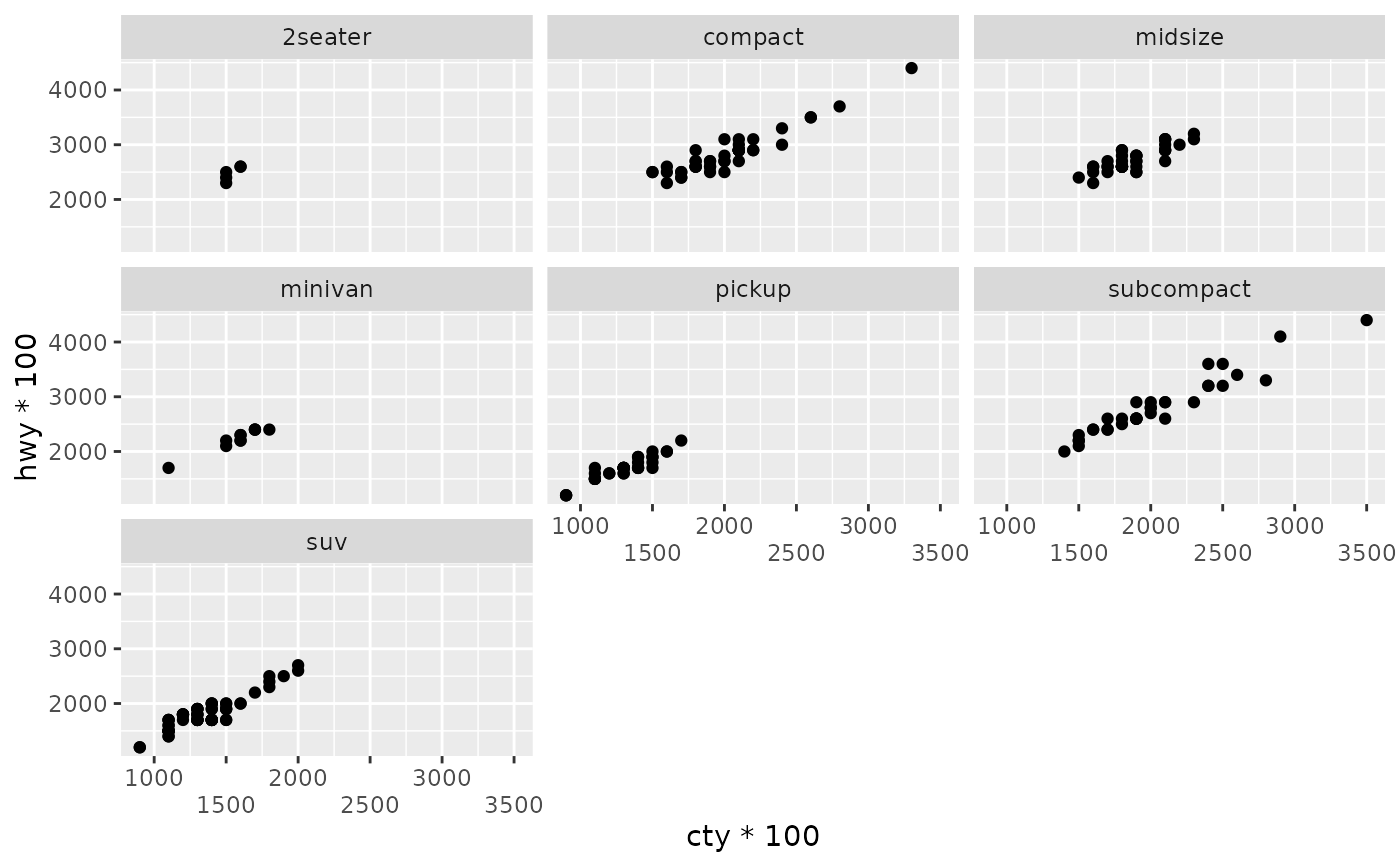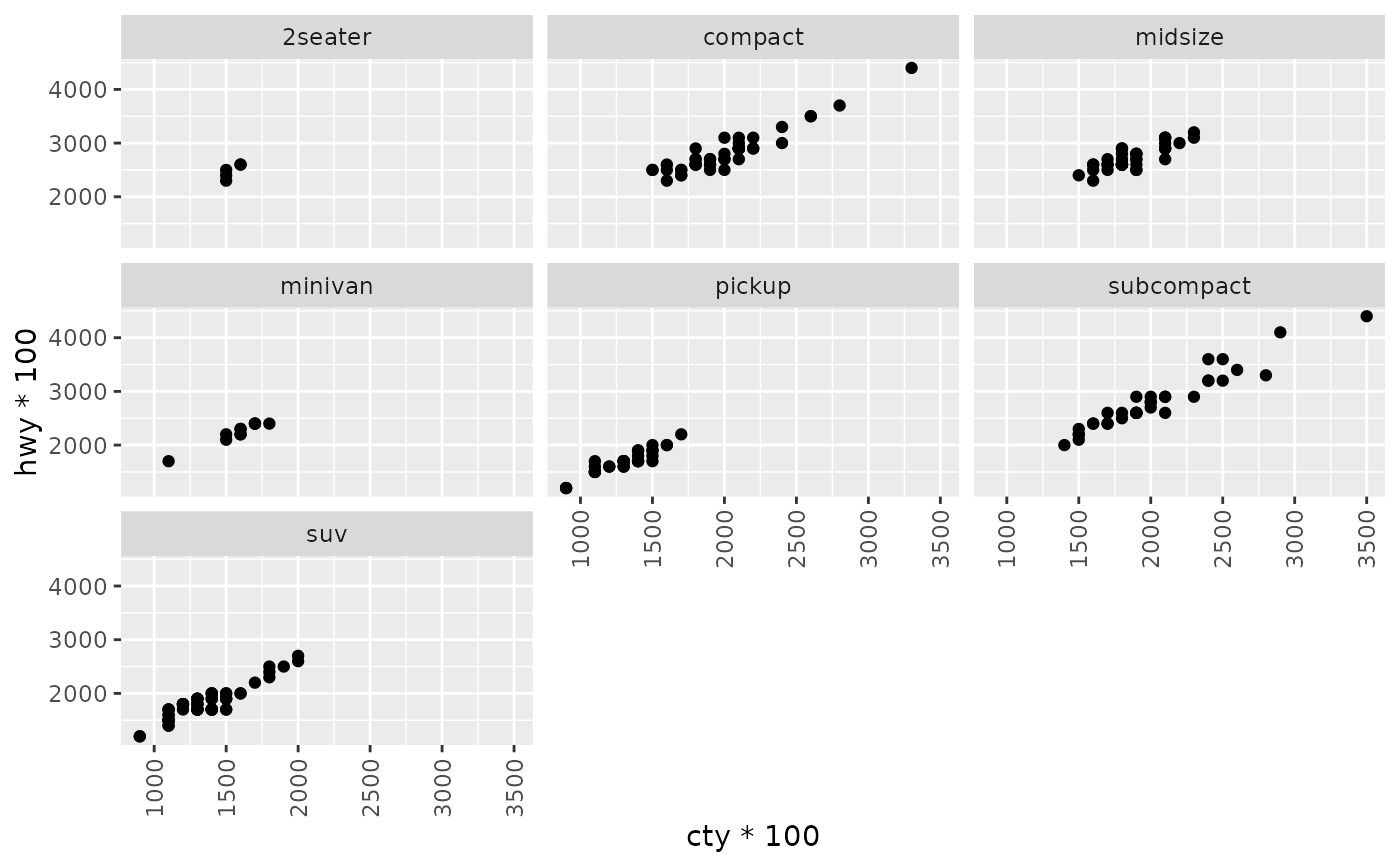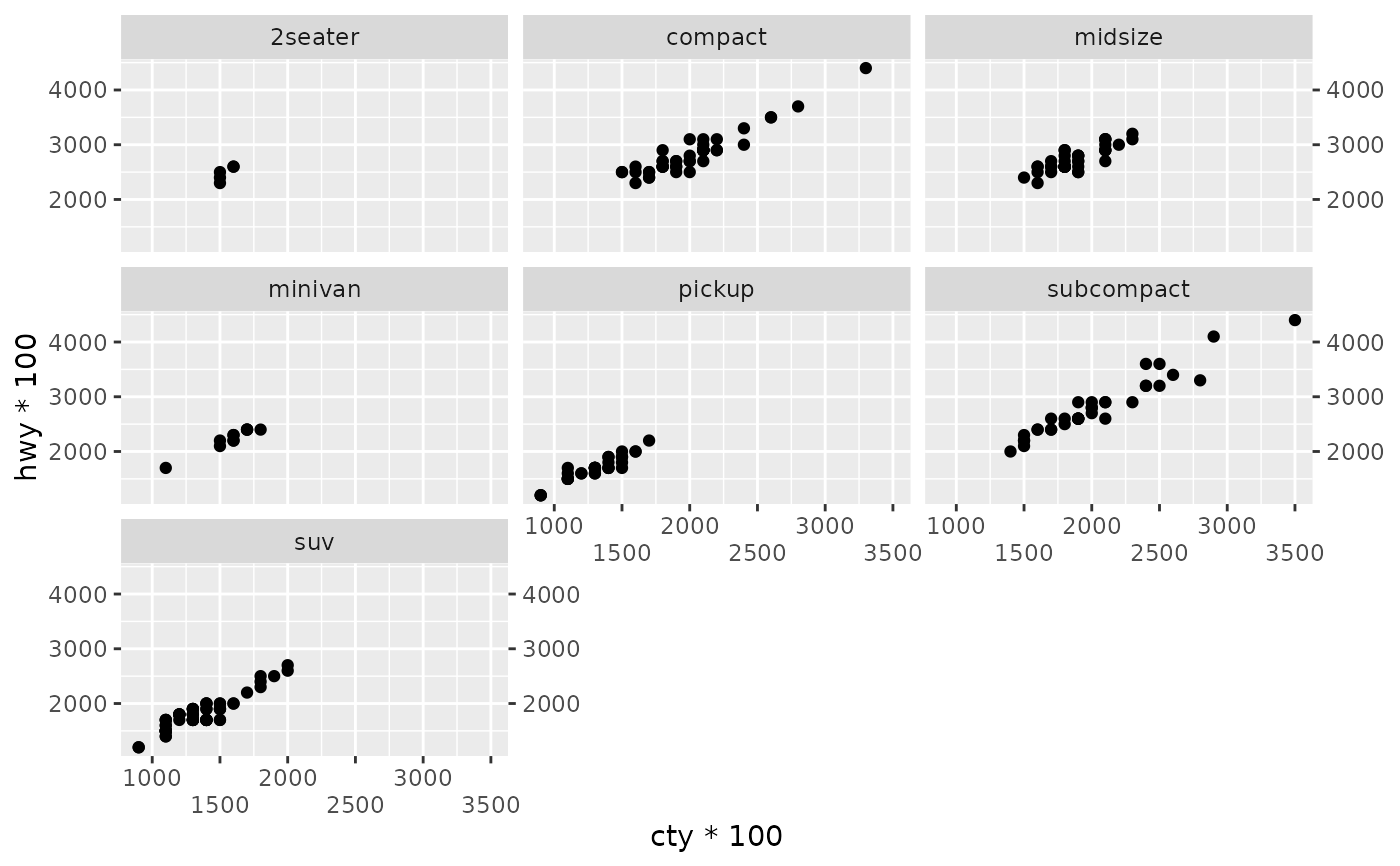Axis guides are the visual representation of position scales like those created with scale_(x|y)_continuous() and scale_(x|y)_discrete().

## Usage

guide_axis(
title = waiver(),
check.overlap = FALSE,
angle = NULL,
n.dodge = 1,
order = 0,
position = waiver()
)

## Arguments

title

A character string or expression indicating a title of guide. If NULL, the title is not shown. By default (waiver()), the name of the scale object or the name specified in labs() is used for the title.

check.overlap

silently remove overlapping labels, (recursively) prioritizing the first, last, and middle labels.

angle

Compared to setting the angle in theme() / element_text(), this also uses some heuristics to automatically pick the hjust and vjust that you probably want.

n.dodge

The number of rows (for vertical axes) or columns (for horizontal axes) that should be used to render the labels. This is useful for displaying labels that would otherwise overlap.

order

Used to determine the order of the guides (left-to-right, top-to-bottom), if more than one guide must be drawn at the same location.

position

Where this guide should be drawn: one of top, bottom, left, or right.

## Examples

# plot with overlapping text
p <- ggplot(mpg, aes(cty * 100, hwy * 100)) +
geom_point() +
facet_wrap(vars(class))

# axis guides can be customized in the scale_* functions or
# using guides()
p + scale_x_continuous(guide = guide_axis(n.dodge = 2))p + guides(x = guide_axis(angle = 90))# can also be used to add a duplicate guide
p + guides(x = guide_axis(n.dodge = 2), y.sec = guide_axis())